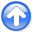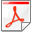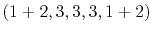Basic operators and adjointsNext: Nearest-neighbor normal moveout (NMO) Up: FAMILIAR OPERATORS Previous: The basic low-cut filter

Smoothing with box and triangle

Simple smoothing'' is a common application of filtering. A smoothing filter is one with all positive coefficients. On the time axis, smoothing is often done with a single-pole damped exponential function. On space axes, however, people generally prefer a symmetrical function. We begin with rectangle and triangle functions. When the function width is chosen to be long, then the computation time can be large, but recursion can shorten it immensely.

The inverse of any polynomial reverberates forever, although it might drop off fast enough for any practical need. On the other hand, a rational filter can suddenly drop to zero and stay there. Let us look at a popular rational filter, the rectangle or box car'':(29)

The filter of equation (29) gives a moving average under a rectangular window. It is a basic smoothing filter. A clever way to apply it is to move the rectangle by adding a new value at one end while dropping an old value from the other end. This approach is formalized by the polynomial division algorithm, which can be simplified, because so many coefficients are either one or zero. To find the recursion associated with, we identify the coefficient ofin. The result is:(30)

This approach boils down to the program which is so fast it is almost free!
api/c/triangle.c
 tmp2 = tmp + 2*nb; wt = 1.0/(2*nb-1); for (i=0; i < nx; i++) { x[o+i*d] = (tmp[i+1] - tmp2[i])*wt; }
Its last line scales the output by dividing by the rectangle length. With this scaling, the zero-frequency component of the input is unchanged, while other frequencies are suppressed.

Triangle smoothing is rectangle smoothing done twice. For a mathematical description of the triangle filter, we simply square equation (29). Convolving a rectangle function with itself many times yields a result that mathematically tends toward a Gaussian function. Despite the sharp corner on the top of the triangle function, it has a shape remarkably similar to a Gaussian. Convolve a triangle with itself and you see a very nice approximation to a Gaussian (the central limit theorem).

With filtering, end effects can be a nuisance, especially on space axes. Filtering increases the length of the data, but people generally want to keep input and output the same length (for various practical reasons), especially on a space axis. Suppose the five-point signalis smoothed using the boxconv() program with the three-point smoothing filter. The output ispoints long, namely,. We could simply abandon the points off the ends, but I like to fold them back in, getting instead. An advantage of the folding is that a constant-valued signal is unchanged by the smoothing. Folding is desirable because a smoothing filter is a low-pass filter that naturally should pass the lowest frequencywithout distortion. The result is like a wave reflected by a zero-slope end condition. Impulses are smoothed into triangles except near the boundaries. What happens near the boundaries is shown in Figure 7.

triend
Figure 7.
Edge effects when smoothing an impulse with a triangle function. Inputs are spikes at various distances from the edge.At the side boundary is only half a triangle, but it is twice as tall.

Why this end treatment? Consider a survey of water depth in an area of the deep ocean. All the depths are strongly positive with interesting but small variations on them. Ordinarily we can enhance high-frequency fluctuations by one minus a low-pass filter, say. If this subtraction is to work, it is important that thetruly cancel thenear zero frequency.

Figure 7 was derived from the routine triangle().

api/c/triangle.c
 tmp1 = tmp + nb; tmp2 = tmp + 2*nb; wt = 1.0/(nb*nb); for (i=0; i < nx; i++) { x[o+i*d] = (2.*tmp1[i] - tmp[i] - tmp2[i])*wt; }Basic operators and adjointsNext: Nearest-neighbor normal moveout (NMO) Up: FAMILIAR OPERATORS Previous: The basic low-cut filter

2014-09-27